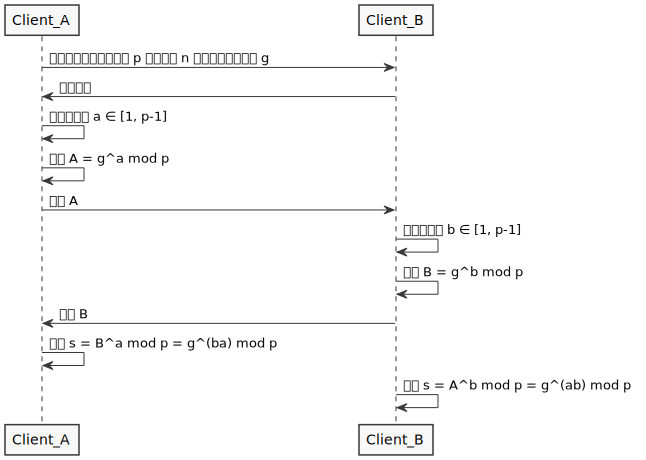# Diffie–Hellman 密钥协商算法详解

## 解决的问题

DH 算法可以在一个不安全的信道上建立安全连接，从而解决的不安全信道上信息安全交换的问题。

## 算法流程与数学原理

DH 算法通过公共信道交换一个信息，就可以创建一个可以用于在公共信道上安全通信的共享秘密（shared secret）。

1. Client_A 与 Client_B 确定算法协商使用质数 p 的整数模 n 乘法群以及其原根 g
2. Client_A 生成随机数 $a \in [1, p-1]$，计算 $A = g^a \mod p$，将 A 发送给 Client_B
3. Client_B 生成随机数 $b \in [1, p-1]$，计算 $B = g^b \mod p$，将 B 发送给 Client_A
4. Client_A 计算 $s = B^a \mod p = g^{ba} \mod p$
5. Client_B 计算 $s = A^b \mod p = g^{ab} \mod p$### Client_A 与 Client_B 算出 s 相等的数学证明

\begin{aligned}
g^b \mod p &= B \
g^b &= kp + B, (k \in N) \
B &= g^b - kp, (k \in N)
\end{aligned}

\begin{aligned}
s &= B^a \mod p \
&= (g^b - kp)^a \mod p \
&= (\binom{a}{0}g^{ba}(-kp)^0 + \binom{a}{1}g^{b(a-1)}(-kp) + \binom{a}{2}g^{b(a-2)}(-kp)^2 + … + \binom{a}{a}g^0(-kp)^a) \mod p \
\end{aligned}

\begin{aligned}
s &= (\binom{a}{0}g^{ba}(kp)^0) \mod p \
&= g^{ab} \mod p
\end{aligned}

### g 的取值范围

DH 算法定义 质数 p 的整数模 n 乘法群以及其原根 g，参考关于原跟整数模n乘法群的数学定义可以知道，原根 g 的取值范围其实是通过 p 计算得出，且范围一定在 [1, p-1] 内。

### a, b 的取值范围

$a = (p-1)j + k \quad (j \in N, k \in [1, p-1])$

$g^a$ 可以做如下变换：

\begin{aligned}
g^a &= g^{(p-1)j+k} \
&= g^{(p-1)j}\cdot g^k \
&= (g^{(p-1)j} \mod p ) \cdot (g^k \mod p) \
&= ((g^{(p-1)})^j \mod p ) \cdot (g^k \mod p) \
&= ((g^{(p-1)} \mod p)^j \mod p ) \cdot (g^k \mod p) \
&= ((1)^j \mod p ) \cdot (g^k \mod p) \
&= (1 \mod p ) \cdot (g^k \mod p) \
&= 1 \cdot (g^k \mod p) \
&= g^k \mod p \
\end{aligned}

• 模除中的恒等式乘法分配律幂运算
• 费马小定理，对于整数 g，质数 p，有 $g^p \mod p = g \mod p$。若 g 不是 p 的倍数，有 $g^{p-1} \mod p = 1$

\begin{aligned}
A &= { g^a, a \in N, a \geq p } \
B &= { g^a, a \in N, a \in [1, p-1] } \
A &= B
\end{aligned}

## 安全性分析

p ✔️ ✔️ ✔️
g ✔️ ✔️ ✔️
a ✔️
b ✔️
A = g^a mod p ✔️ ✔️ ✔️
B = g^b mod p ✔️ ✔️ ✔️
s = A^b mod p ✔️
s = B^a mod p ✔️

### p 的安全范围

1. Client_A 计算 $A = 3^2 \mod 7 = 2$，将 A 发送给 Client_B
2. Client_B 计算 $B = 3^5 \mod 7 = 5$，将 B 发送给 Client_A
3. Client_A 计算 $s = 5^2 \mod 7 = 4$
4. Client_B 计算 $s = 2^5 \mod 7 = 4$

$a$ $g^a \mod p$
1 3
2 2
3 6
4 4
5 5
6 1

g 有时被选择成 G 的 q 阶子群的生成元，而不是 G 本身的生成元，这样 $g^a$ 的勒让德符号将不会显示出 a 的低位。

### 身份验证

DH 密钥交换本身并没有提供通讯双方的身份验证服务，因此它很容易受到中间人攻击。 一个中间人在信道的中央进行两次 DH 密钥交换，一次和 A 另一次和 B，就能够成功的向 A 假装自己是B，反之亦然。中间人者可以解密（读取和存储）任何一个人的信息并重新加密信息，然后传递给另一个人。通常需要一个能够验证通讯双方身份的机制来防止这类攻击，比如一个公钥基础设施。